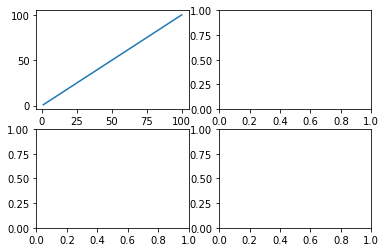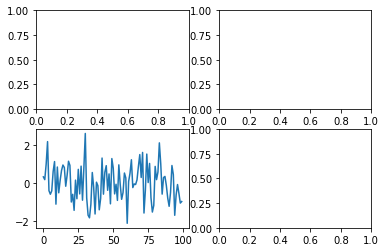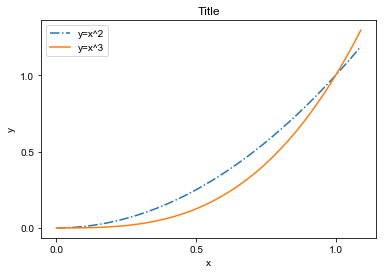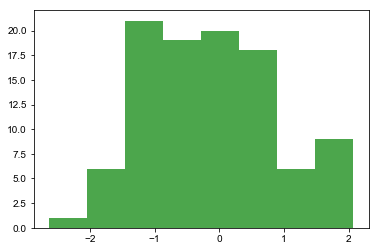# Matplotlib可视化库的简单使用

1通过subplot创建单个子图

```#分成2*2的矩阵区域
plt.subplot(221)
plt.plot(nums,nums)
plt.subplot(222)
plt.plot(nums,-nums)
plt.subplot(212)
plt.plot(nums,nums**2)
plt.show()```

2通过subplots创建多个子图

```#生成包含1～100之间所有整数数组
nums = np.arange(1,101)
#分成2*2的矩阵区域 返回子图数组axes
fig,axes = plt.subplots(2,2)
axes[0,0].plot(nums,nums)
plt.show()```

```fig = plt.figure()
#添加子图
#在子图上作图
random_arr = np.random.randn(100)
#默认是在最后一次使用subplot的位置上作图 即编号为3的位置
plt.plot(random_arr)
plt.show()```

4添加各类标签

title():设置当前轴的标题

xlabel():设置当前图形X轴的标签名称

ylabel():设置当前图形Y轴的标签名称

xticks():指定X轴刻度的数目与取值

yticks():指定Y轴刻度的数目与取值

xlim():设置或获取当前图形X轴的范围

ylim():设置或获取的当前图形Y轴的范围

legend():在轴上放置一个图例

```import numpy as np
data = np.arange(0,1.1,0.01)
plt.title('Title')
plt.xlabel('x')
plt.ylabel('y')
#设置x和y轴的刻度
plt.xticks([0,0.5,1])
plt.yticks([0,0.5,1.0])
plt.plot(data,data**2,lineStyle='-.')
plt.plot(data,data**3)
plt.legend(['y=x^2','y=x^3'])
plt.show(```

```#windos下
from pylab import mpl
mpl.reParams['font,sans-serif'] = ['SimHei']
#mac下
from pylab import mpl
mpl.rcParams['font.sans-serif'] = ['Arial Unicode MS']```

`mpl.rcParams['axes.unicode_minus'] = False`

bar绘制条形图  pie:绘制饼图 scatter:绘制散点图 plot:绘制折线图 boxplot:绘制箱型图 hist:绘制直方图 barh 绘制水平条形图

```plt.hist(np.random.randn(100),bins=8,color='g',alpha=0.7)
plt.show()```

```x = np.arange(51)
#创建另一个数组  表示很y轴数据
y = np.random.rand(51)*10
plt.scatter(x,y,marker='p')
plt.show()```

```import matplotlib.pyplot as plt
plt.rcParams['font.sans-serif'] = ['Arial Unicode MS']  #正常显示中文标签
area = jn['山东省,济宁市'].values
plt.figure(figsize=(12,6))
x_num = range(0,len(area))
y_dis = [i+0.3 for i in x_num]
plt.bar(x_num,area,colors='g',width=.3,label='总面积',labels='')
plt.show()```

### 手机扫码访问

•